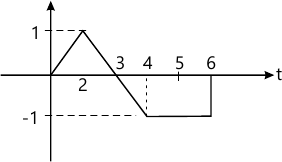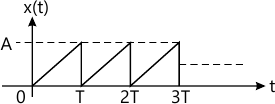MORE IN Signals & Systems
Total marks: --
Total time: --
INSTRUCTIONS
(1) Assume appropriate data and state your reasons
(2) Marks are given to the right of every question
(3) Draw neat diagrams wherever necessary

1 (a) Determine whether the discrete time signal $x(n)= \cos \left ( \dfrac {\pi n}{5} \right ) \sin \left ( \dfrac {\pi n}{3} \right )$ is periodic. If periodic, find the fundamental period.
4 M
1 (b)

Determine whether the signal shown in Fig. Q1(b) is a power signal or energy signal. Justify your answer and further determine its energy/power.6 M
1 (c) Given the signal x(n)=(6-n){u(n)-u(n-6)} make a sketch of x(n), y1(n)=x(4-n) and y2(n)=x(2n-3).
4 M
1 (d) Find and sketch the following signals and their derivatives:
i) x(t)=u(t)-u(t-a); a>0
ii) y(t)=t[u(t)-u(t-a)];a?0
6 M

2 (a) The impulse response of discrete LTI system is give by, h(n)=u(n+1)-u(n-4). The system is excited by the input signal x(n)=u(n)-2u(n-2)+u(u-4). Obtain the response of the system y(n)=x(n)×h(n) and plot the same.
7 M
2 (b) Given x(t)=t 0
7 M
2 (c) Show that:
i) x(t)× h(t) = h(t)× x(t)
ii) {x(n)× h1(n)} × h2(n)=x(n)×{h1(n)×h2(n)}.
6 M

3 (a) Solve the difference equation, y(n)-3y(n-1)-4y(n-2)=x(n) with x(n)=4nu(n). Assume that the system is initially relaxed.
6 M
3 (b) Draw the direct form I and direct form II implementation for $i) \ y(n) - \dfrac {1}{2} y(n-1)-y(n-3)= 3x(n-1)+ 2x(n-2) \\ ii) \ \dfrac {d^2 y(t)}{dt^2}+ 5 \dfrac {dy (t)}{dt}+ 4y(t)= \dfrac {dx (t)}{dt}$
10 M
3 (c) Define causality. Derive the necessary and sufficient conditions for a discrete LTI system to be causal in terms of the impulse response.
4 M

4 (a) Determine the DTFS coefficients of. $x(n)= 1+\sin \left \{ \dfrac {1}{12} \pi n + \dfrac {3\pi}{8} \right \}$
6 M
4 (b)

Find the exponential Fourier series of the waveform shown in Fig Q4(b).8 M
4 (c) Explain the Direchlet conditions for the existence of Fourier series.
6 M

5 (a) Find the DTFT of the signal x(n) given by x(n)=u(n)-u(n-N); where N is any +ve integer. Determine the magnitude and phase components and draw the magnitude spectrum for N=5.
10 M
5 (b) Determine the Fourier transform of the following signals: i) x(t)=e-3tu(t-1)
ii) x(t)=e-a|t
10 M

6 (a) Determine the frequency response and the impulse response for the system described by the differential equation, $\dfrac {d^2 y(t)}{dt^2} + \dfrac {dy(t)}{dt}+ 6y(t)= \dfrac {-d}{dt}x(t)$
10 M
6 (b) Determine the Nyquist sampling rate and Nyquist sampling interval for, $i) \ x(t)=1+\cos 2000 \pi t + \sin 4000 \pi t \\ ii) \ x(t)= \left [ \dfrac {\sin (4000 \pi t)}{\pi t} \right ]^2$
6 M
6 (c) Explain briefly, the reconstruction of continuous time signals with zero order hold.
4 M

7 (a) Find the z-transform of the following and indicate the region of convergence:
1) x(n)=an cos?0 (n-2)u(n-2)
ii) x(n)=n(n+1)anu(n)
10 M
7 (b) Find the inverse z-transform of the following: $i) \ x(z)= \dfrac {z^4 +z^2}{z^2-\frac {3}{4}z+\frac {1}{8}}; |z|>\dfrac {1}{2} by$ Partial fraction expansion method. $ii) \ x(z)= \dfrac {1-az^{-1}}{z^{-1}-a}; z>\dfrac {1}{a}$ by long division method.
10 M

8 (a) A discrete LTI system is characterized by the different equation, y(n)=y(n-1)+y(n-2)+x(n-1)
Find the system function H(z) and indicate the ROC if the system, i) Stable ii) Causal. Also determine the unit sample response of the stable system.
10 M
8 (b) Solve the following difference equation using unilateral z-transform. $y(n) - \dfrac {7}{12} y(n-1)+ \dfrac {1}{12}y(n-2)=x(n), \ for \ n\ge 0, \ with \ initial \ conditions \ y(-1)=2, y(-2)=4, \ and \ x(n)= \left ( \dfrac {1}{5} \right )^n u(n)$
10 M

More question papers from Signals & Systems